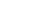Thank you!
Our representative will contact you shortly
Error occurred while submitting data. Please try again after some time.
Fill in the details below

We will call you back as soon as possibleCalculators
Apply Now

# Calculators

Get an approximate figure for the total monthly instalment payments along with a complete break-up of the home loan.

#### Home Loan Eligibility CalculatorOur free online home Loan Eligibility Calculator to get an estimate of the maximum home loan amount you may be eligible to get

Calculate home loan eligibility

#### Home Loan EMI CalculatorGet an approximate figure for the total monthly instalment payments along with a complete break-up of the home loan.

Calculate Home Loan EMI

#### LAP EMI CalculatorGet an approximate figure for the total monthly instalment payments along with a complete break-up of the loan against property.

Calculate LAP EMI

#### APR CalculatorUse our APR Calculator to know the actual cost or annual percentage rate of your loan

Calculate APR

#### Home Loan Prepayment CalculatorList the Home Loan Prepayment Calculator just above the mentioned screenshot calculators.

Home Loan Prepayment Calculator

## What is EMI?

Whether it is a home loan or a loan against property, or any other type of loan, borrowers always avail loans for a specific purpose. A loan that has been taken will have to be repaid with interest. The most common form of repayment is the deduction of a predetermined amount every month from the borrower’s bank account towards the principal and interest of the loan.

These standardised deductions are known as Equated Monthly Instalments or EMIs. The EMI amount depends upon 4 factors – the loan type (floating or fixed), the repayment tenure, the interest rate and the loan amount or principal.

In the case of a floating loan type, there is an additional factor known as the “Rest”.

The EMI amount is directly proportional to the principal borrowed with all the other parameters remaining constant. The same holds true for the interest rate and the EMI, other factors remaining constant. The repayment tenure and the EMI are inversely proportional to each other.

## How to use a Loan EMI calculator

A Loan EMI calculator is a tool that enables borrowers to calculate the amount that has to be repaid every month towards the loan. It can be used to calculate EMIs against all types of loans.

Every loan EMI calculator comprises 3 variables – the principal (P), the interest rate (R) and the repayment tenure (N). The EMI is calculated the following way

EMI = [P*R*(1+R) ^N]/ [(1+R) ^N-1]

We can understand a loan EMI calculator better with the help of an example

Let us suppose that a borrower has taken a loan of Rs.10, 00,000 for 5 years at a 10% annual interest rate.

The principal amount is 10, 00,000. The annual interest rate divided into months is 10/100/12 and the repayment tenure expressed in months is 60 months.

So, EMI = [10, 00,000 * (10%/12) * (1+(10%/12)) ^60]/ [(1+(10%/12)) ^60-1] - please note that since the interest rate (10%) is calculated on a per annum basis, it needs to be divided by 12 in the formula.

EMI = Rs. 21,247/-

## Floating rate EMI calculation

Fullerton Grihashakti only offers loans at floating rates of interest on home loans and loans against property.

An interest rate that is variable and changes in the course of the repayment tenure of a loan is known as a floating rate of interest. Floating rates of interest are periodically adjusted according to market conditions.

The change in the floating rate of interest is linked to the FIHFC Retail Prime Lending Rate.

## Types of EMI Calculators

Fullerton Grihashakti offers 4 types of EMI calculators to its customers – a Home Loan Calculator, Loan against Property Calculator, Moratorium Calculator and Home Loan Eligibility Calculator.

Moratorium EMI calculator

Recognising the difficulty of repaying loans while the Covid-19 pandemic rages, the RBI had declared a moratorium on all term loans in 2020. Using the Fullerton Grihashakti Moratorium EMI calculator, all borrowers can estimate how much their loan tenure or EMI will change after they apply the RBI moratorium to their loans.

Home Loan EMI Calculator

Borrowers can use this loan EMI calculator to accurately calculate their home loan EMI by punching in their home loan amount, amount of interest and repayment tenure.

Loan against Property EMI calculator

Similar to the above calculator, the loan against property EMI calculator allows borrowers to accurately gauge their LAP EMI based on their principal amount, interest rates and repayment tenure.

Home Loan Eligibility Calculator

With the help of the following variables – monthly expenses, the value of the property and monthly income, Fullerton Grihashakti helps borrowers calculate how much loan they could get.